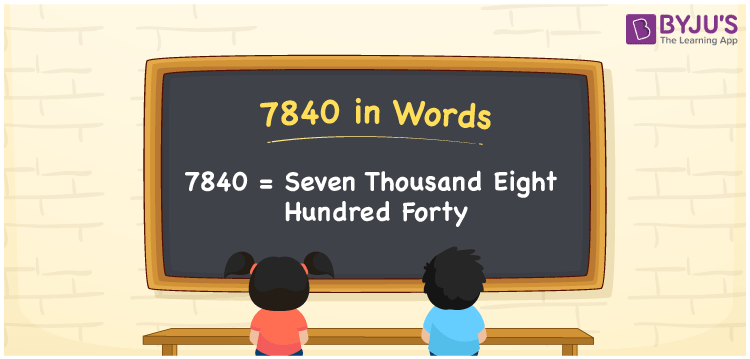# 7840 in words

7840 in words is written as Seven Thousand Eight Hundred and Forty. In 7840, 7 has a place value of thousand, 8 is in the place value of hundred and 4 is in the place value of ten. The article on Place Value gives more information. The number 7840 is used in expressions that relate to money, distance, length, area and many more. For example, “Can you transfer Seven Thousand Eight Hundred and Forty rupees to my account?”

 7840 in words Seven Thousand Eight Hundred and Forty Seven Thousand Eight Hundred and Forty in Numbers 7840

## 7840 in English Words## How to Write 7840 in Words?

We can convert 7840 to words using a place value chart. The number 7840 has 4 digits, so let’s make a chart that shows the place value up to 4 digits.

 Thousands Hundreds Tens Ones 7 8 4 0

Thus, we can write the expanded form as:

7 × Thousand + 8 × Hundred + 4 × Ten + 0 × One

= 7 × 1000 + 8 × 100 + 4 × 10 + 0 × 1

= 7840.

= Seven Thousand Eight Hundred and Forty.

7840 is the natural number that is succeeded by 7839 and preceded by 7841.

7840 in words – Seven Thousand Eight Hundred and Forty.

Is 7840 an odd number? – No.

Is 7840 an even number? – Yes.

Is 7840 a perfect square number? – No.

Is 7840 a perfect cube number? – No.

Is 7840 a prime number? – No.

Is 7840 a composite number? – Yes.

## Solved Example

1. Write the number 7840 in expanded form

Solution: 7 x 1000 + 8 x 100 + 4 x 10 + 0 x 1

Or Just 7 x 1000 + 8 x 100 + 4 x 10

We can write 7840 = 7000 + 800 + 40 + 0

= 7 x 1000 + 8 x 100 + 4 x 10 + 0 x 1.

## Frequently Asked Questions on 7840 in words

Q1

### How to write the number 7840 in words?

7840 in words is written as Seven Thousand Eight Hundred and Forty.
Q2

### Is 7840 divisible by 3?

No. 7840 is not divisible by 3.
Q3

### Is 7840 divisible by 10?

Yes. 7840 is divisible by 10.